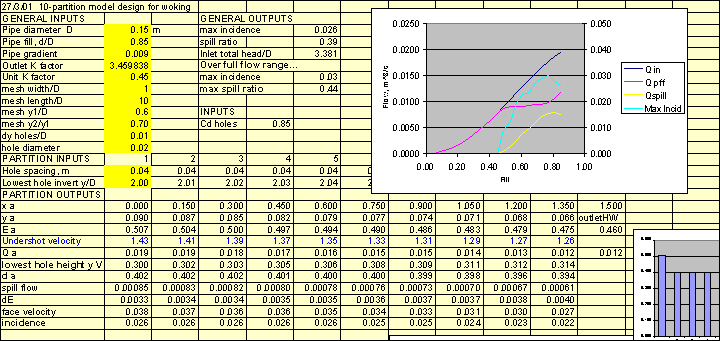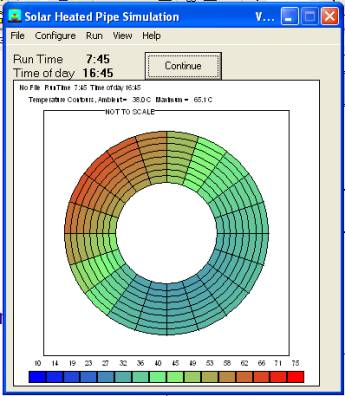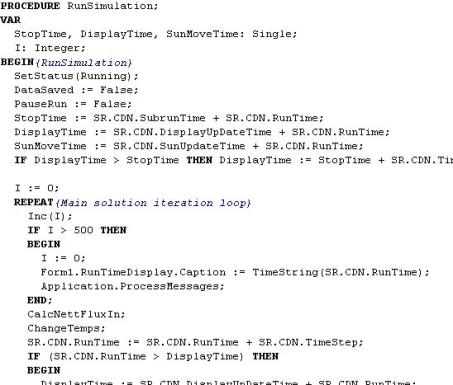# Mathematical Modelling

Mathematical models require a deep understanding of the physics and the ability to implement the underlying equations.  Mosts tasks are solved using spreadsheets, an engineering (Newton’s method) equation solver, or a programming language (Fortran, Pascal, Delphi, Visual Basic).  Many models are spreadsheet-based so that they are portable and accessible for the client to run if they choose.

INDEX

Wastewater treatment device hydraulics

## Wastewater treatment device hydraulics

For associated simulation and experimental work see “wastewater treatment” under Fluid Mechanics.  A combination of numerical modeling (CFD), mathematical modeling and experimental work was valuable to this project.Back to Index

## Kinematic model of steering shaft.

This model was created to predict the velocity ratio through a multiple-jointed shaft with generalised geometry.  It applies matrix methods to spatial transforms as used in robotics.  Used in the automotive industry.  Such prediction can be done today with advanced commercial CAD packages, but this special spreadsheet tool is still more convenient in many cases.Back to Index

## Thermal hydraulic system

This model, for an American client, was used to predict the performance of a domestic hot water system using a venturi-driven hot water circulation concept.Back to Index

This is a simple example of a mechanism (Watt’s linkage) solved using a Newton’s method equation solver.Back to Index

## Transient temperature field in a pipeline wall exposed to the sun

This is an example of a finite element model coded in Delphi™, used to predict the wall temperature in pipelines given latitude, pipe direction etc.Back to Index